# Cryptographic Hashing BlockcipherBased Constructions Revisited Tom Shrimpton Portland

• Slides: 40
Download presentationCryptographic Hashing: Blockcipher-Based Constructions, Revisited Tom Shrimpton Portland State University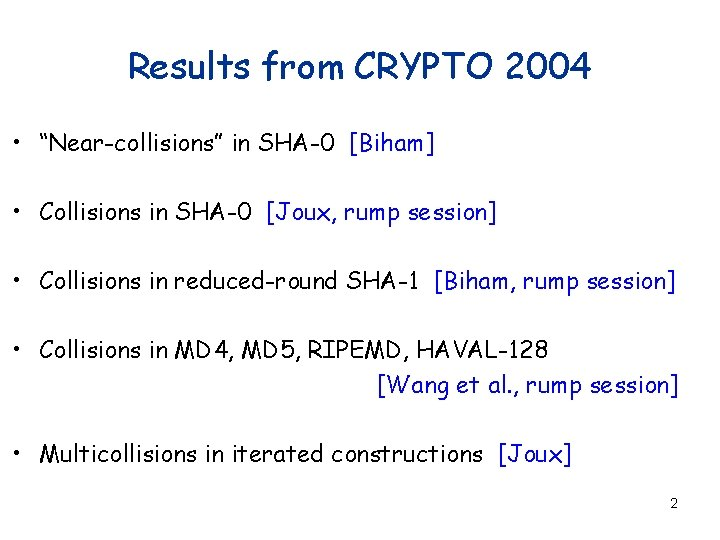Results from CRYPTO 2004 • “Near-collisions” in SHA-0 [Biham] • Collisions in SHA-0 [Joux, rump session] • Collisions in reduced-round SHA-1 [Biham, rump session] • Collisions in MD 4, MD 5, RIPEMD, HAVAL-128 [Wang et al. , rump session] • Multicollisions in iterated constructions [Joux] 2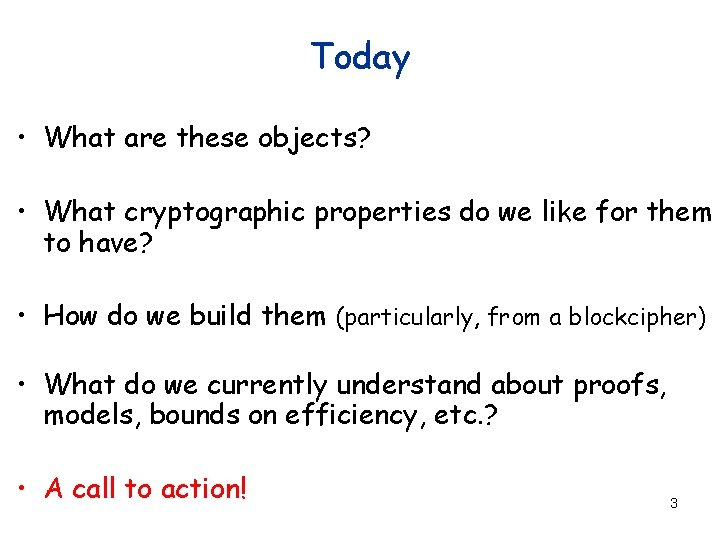Today • What are these objects? • What cryptographic properties do we like for them to have? • How do we build them (particularly, from a blockcipher) • What do we currently understand about proofs, models, bounds on efficiency, etc. ? • A call to action! 3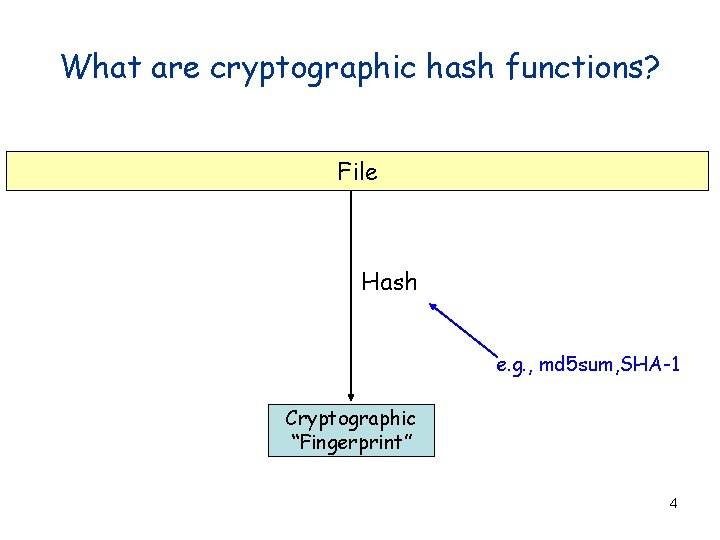What are cryptographic hash functions? File Hash e. g. , md 5 sum, SHA-1 Cryptographic “Fingerprint” 4SHA-1 512 bits M 1 [NIST] . . . M 2 Mm for i = 1 to m do Wt = { A ¬ H 0 i-1; t-th word of Mi ( Wt-3 Å Wt-8 Å Wt-14 Å Wt-16 ) << 1 B ¬ H 1 i-1; C ¬ H 2 i-1; 0 £ t £ 15 16 £ t £ 79 D ¬ H 3 i-1; E ¬ H 4 i-1 for t = 0 to 79 do T ¬ A << 5 + gt (B, C, D) + E + Kt + Wt E ¬ D; D ¬ C; C ¬ B >> 2; B ¬ A; A ¬ T end H 0 i ¬ A + H 0 i-1; H 3 i ¬ D + H 3 i-1; H 1 i ¬ B + H 1 i-1; H 4 i ¬ E + H 4 i-1 end return H 0 m H 1 m H 2 m H 3 m H 4 m H 2 i ¬ C+ H 2 i-1; 160 bits 5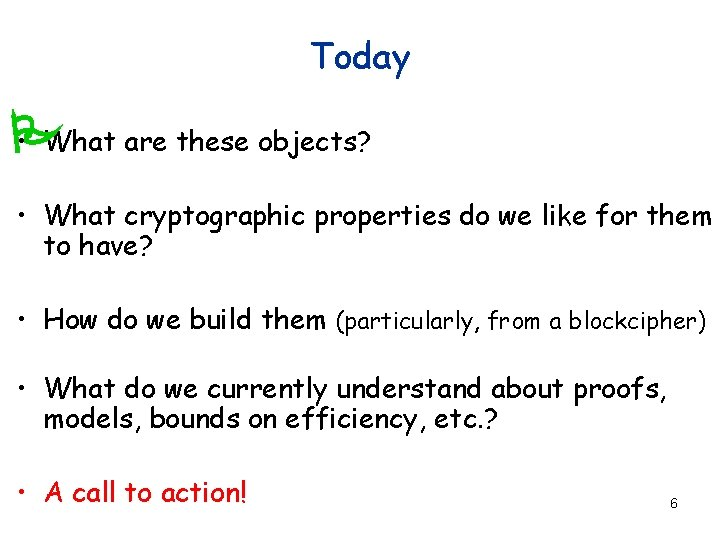Today • What are these objects? P • What cryptographic properties do we like for them to have? • How do we build them (particularly, from a blockcipher) • What do we currently understand about proofs, models, bounds on efficiency, etc. ? • A call to action! 6i nd -pre 2 e er g a m ce n a t sis ? weak ? target str ong ? ? collision ? collis ? ? ? col lisi on res i ? nce ? free n o i collis inversio nce n resist ance ? ? ash h g n stro one-way function resista ? ? ion re sista collision-intractable ? universal one -way hash fu nction ? sta nce ? i e r p g ma e ? is s e r ? collision resistance e c n a t ? 7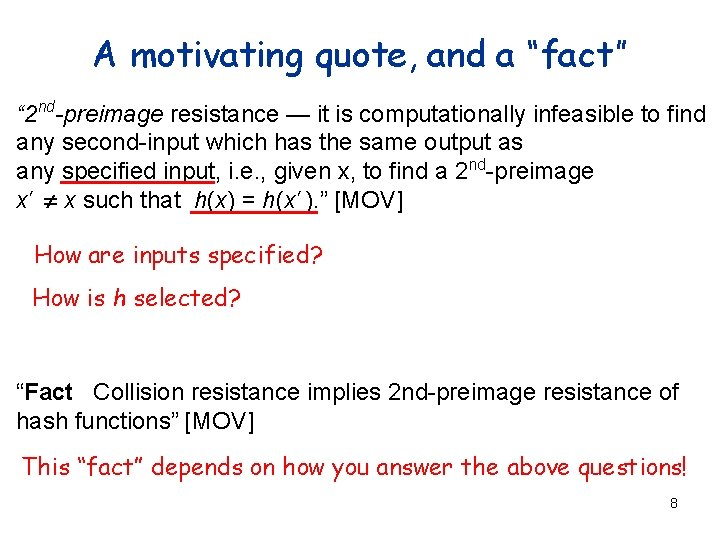A motivating quote, and a “fact” “ 2 nd-preimage resistance — it is computationally infeasible to find any second-input which has the same output as any specified input, i. e. , given x, to find a 2 nd-preimage x’ x such that h(x) = h(x’). ” [MOV] How are inputs specified? How is h selected? “Fact Collision resistance implies 2 nd-preimage resistance of hash functions” [MOV] This “fact” depends on how you answer the above questions! 8A cryptographic property (quite informal) 1. Preimage resistance: given a hash function and given a hash output it is hard to invert that output BAD: H(M) = M mod 701 9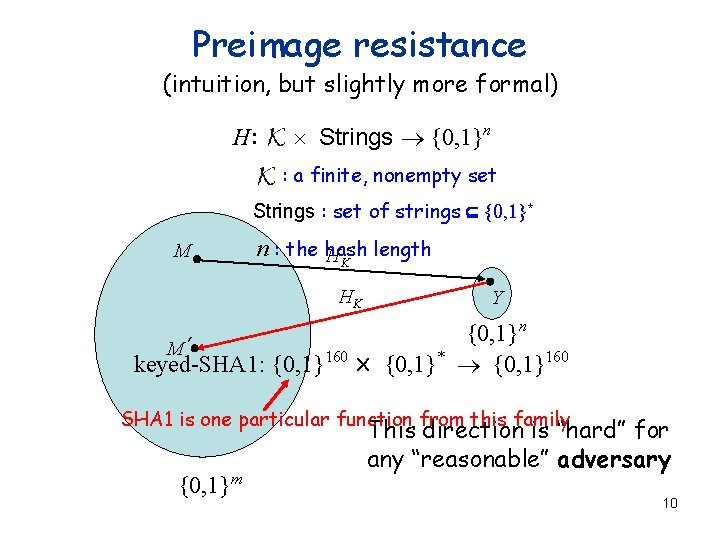Preimage resistance (intuition, but slightly more formal) H: ´ Strings ® {0, 1}n : a finite, nonempty set Strings : set of strings M {0, 1}* n : the hash HK length HK M’ Í keyed-SHA 1: {0, 1}160 Y {0, 1}n ´ {0, 1}* ® {0, 1}160 SHA 1 is one particular function from this family {0, 1}m This direction is “hard” for any “reasonable” adversary 10Preimage resistance: a definition (formal) probabilistic game “name of game” - random key - random domain pt - hash the domain pt - A runs, returns domain pt event: did A win (find preimage)? 11A formal framework [RS 04] Preimage fixed random range point a. Pre fixed key random key e. Pre Every range point is hard to invert Every hash function in the family is hard to invert Pre “a” = “always” “e” = “everywhere” 12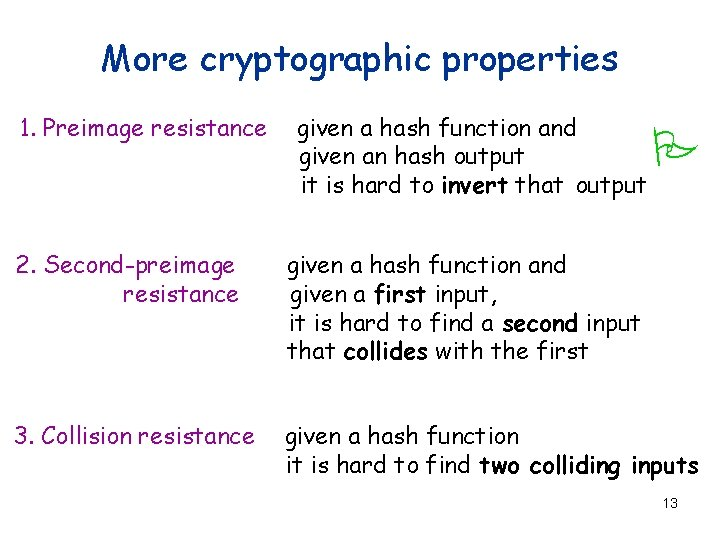More cryptographic properties 1. Preimage resistance given a hash function and given an hash output it is hard to invert that output P 2. Second-preimage resistance given a hash function and given a first input, it is hard to find a second input that collides with the first 3. Collision resistance given a hash function it is hard to find two colliding inputs 13Preimage fixed random range point a. Pre fixed key random key e. Pre Second Preimage fixed random domain point a. Sec fixed key random key e. Sec Collision Also known as UOWHF fixed key random key CollOur results[RS 04] Coll a. Sec e. Sec Provisional Conventional [no arrow] Separation a. Pre e. Pre 15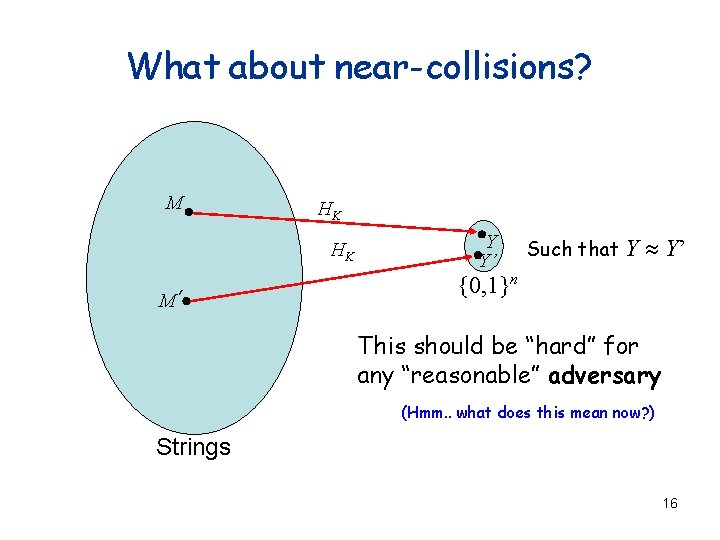What about near-collisions? M HK HK M’ Y Y’ Such that Y » Y’ {0, 1}n This should be “hard” for any “reasonable” adversary (Hmm. . what does this mean now? ) Strings 16Research project #1 Continue definitional work What’s the “right” definition for the task? How do we make it formal? 17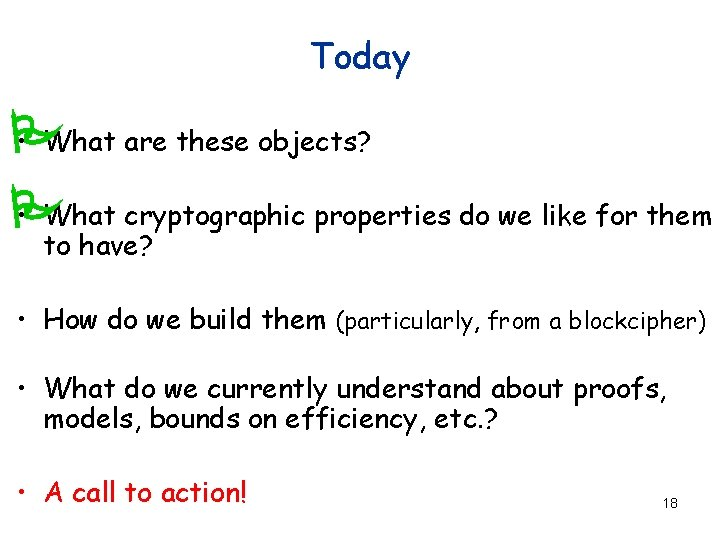Today • What are these objects? P • What cryptographic properties do we like for them P to have? • How do we build them (particularly, from a blockcipher) • What do we currently understand about proofs, models, bounds on efficiency, etc. ? • A call to action! 18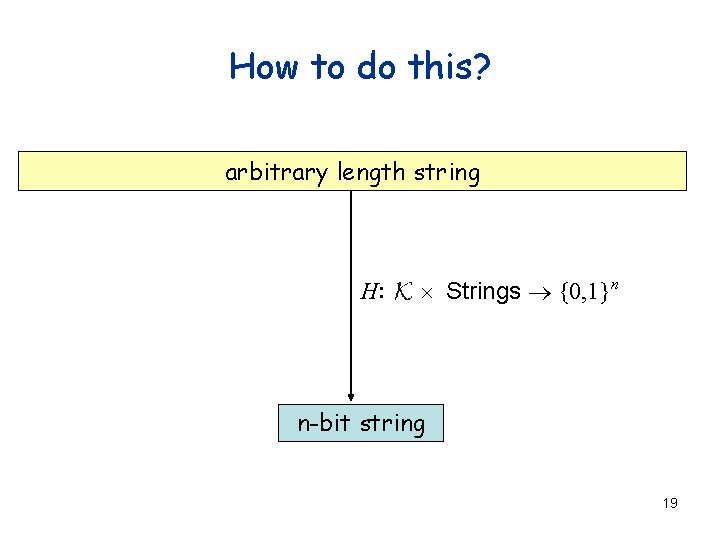How to do this? arbitrary length string H: ´ Strings ® {0, 1}n n-bit string 19Merkle-Damgard construction [Me 89], [Da 89] Compression function M 1 M 3 M 2 n f IV h 1 f h 2 f k k Fixed initial value h 3 = H (M) Chaining value MD Theorem: if f is CR, then so is H 20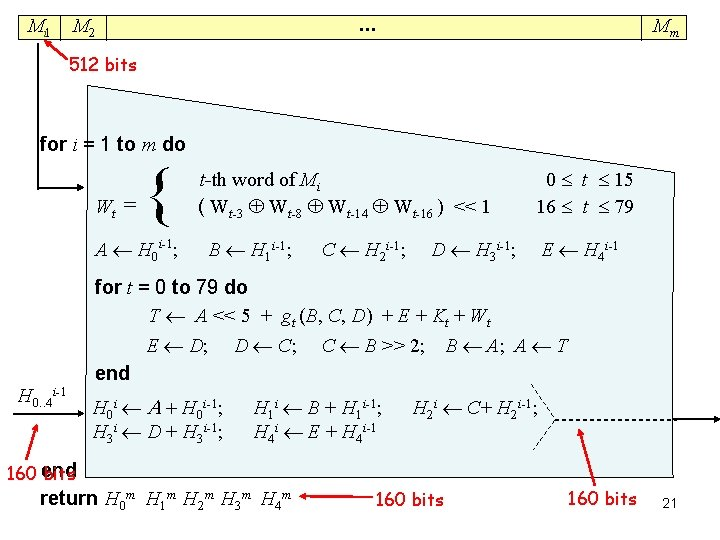Mi 1 . . . M 2 Mm 512 bits for i = 1 to m do Wt = { A ¬ H 0 i-1; t-th word of Mi ( Wt-3 Å Wt-8 Å Wt-14 Å Wt-16 ) << 1 B ¬ H 1 i-1; C ¬ H 2 i-1; 0 £ t £ 15 16 £ t £ 79 D ¬ H 3 i-1; E ¬ H 4 i-1 for t = 0 to 79 do T ¬ A << 5 + gt (B, C, D) + E + Kt + Wt E ¬ D; D ¬ C; C ¬ B >> 2; B ¬ A; A ¬ T end H 0. . 4 i-1 H 0 i ¬ A + H 0 i-1; H 3 i ¬ D + H 3 i-1; H 1 i ¬ B + H 1 i-1; H 4 i ¬ E + H 4 i-1 H 2 i ¬ C+ H 2 i-1; 160 end bits return H 0 m H 1 m H 2 m H 3 m H 4 m 160 bits 21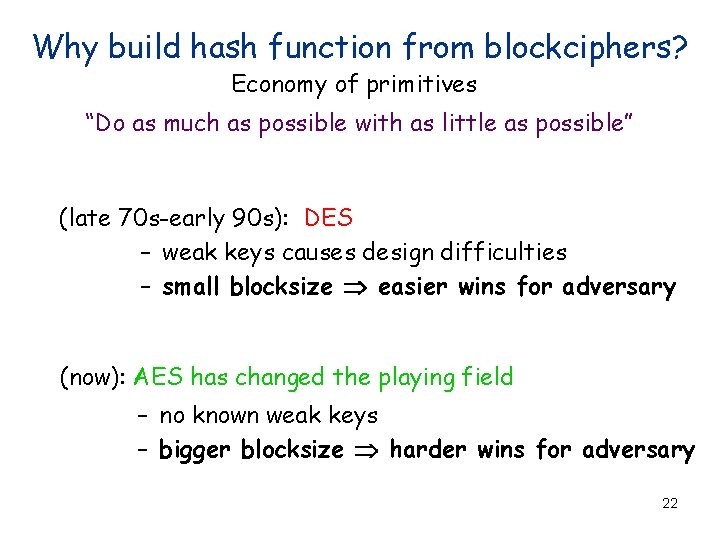Why build hash function from blockciphers? Economy of primitives “Do as much as possible with as little as possible” (late 70 s-early 90 s): DES – weak keys causes design difficulties – small blocksize Þ easier wins for adversary (now): AES has changed the playing field – no known weak keys – bigger blocksize Þ harder wins for adversary 22Blockcipher-based compression function #1 (CBC) [Akl 83] Is this collision-resistant? IV 0 M 0 EK(IV)MÅ EK(0) E K 1 IV K 2 E EK(EK(0)) = EK(EK(0)) 23Attempt #2 [PGV 93] How about this? IV IV Å 1 M IV 1 E 1(1) Å IV E 0(0) M 2Å IV E E IV = IV 24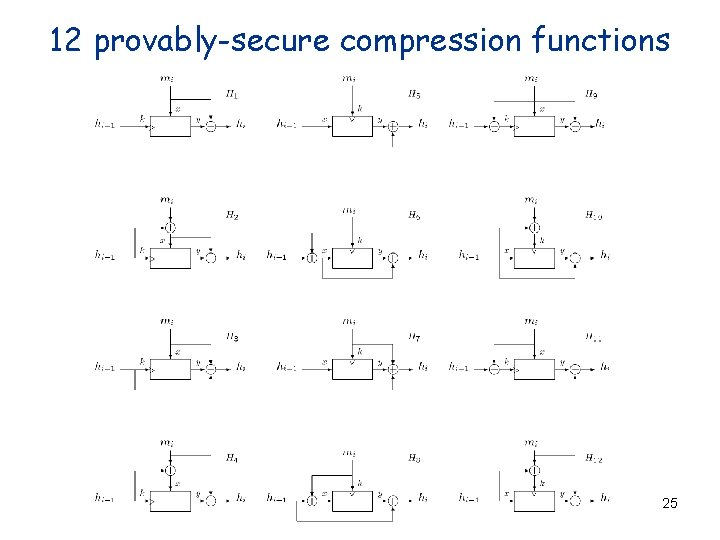12 provably-secure compression functions 25Davies-Meyer compression function [PGV 93], [BRS 02] Mi hi-1 E hi 26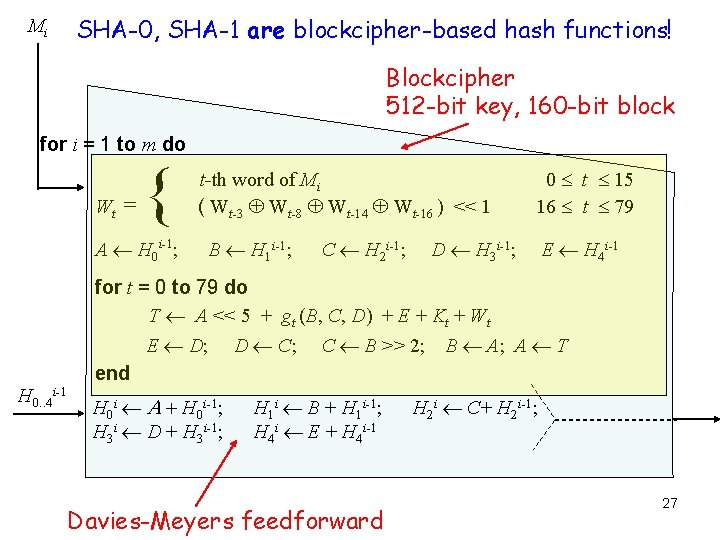Mi SHA-0, SHA-1 are blockcipher-based hash functions! Blockcipher 512 -bit key, 160 -bit block for i = 1 to m do Wt = { A ¬ H 0 i-1; t-th word of Mi ( Wt-3 Å Wt-8 Å Wt-14 Å Wt-16 ) << 1 B ¬ H 1 i-1; C ¬ H 2 i-1; 0 £ t £ 15 16 £ t £ 79 D ¬ H 3 i-1; E ¬ H 4 i-1 for t = 0 to 79 do T ¬ A << 5 + gt (B, C, D) + E + Kt + Wt E ¬ D; D ¬ C; C ¬ B >> 2; B ¬ A; A ¬ T end H 0. . 4 i-1 H 0 i ¬ A + H 0 i-1; H 3 i ¬ D + H 3 i-1; H 1 i ¬ B + H 1 i-1; H 4 i ¬ E + H 4 i-1 Davies-Meyers feedforward H 2 i ¬ C+ H 2 i-1; 27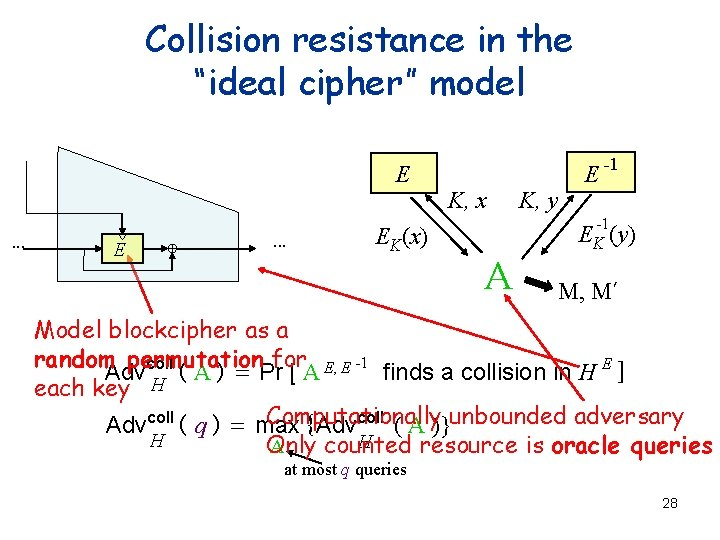Collision resistance in the “ideal cipher” model E. . . EK(x) K, x K, y E -1 -1 EK (y) A M, M’ Model blockcipher as a random permutation for E, E -1 E Advcoll ( A ) = Pr [ A finds a collision in H ] H each key Computationally Advcoll ( q ) = max {Advcoll ( A )}unbounded adversary H H Only counted resource is oracle queries A at most q queries 28Why such a strong model? PRP assumption isn’t enough in general [Simon] Specifically, for each of the 12 there is a PRP that makes collisions easy [Hopwood][Wagner] More importantly, PRP is the wrong tool Security depends on a random, secret key 29Research project #2 Find new models and/or assumptions What properties does a blockcipher need for hashing? How can we abstract them to models/assumptions? Can we prove things? 30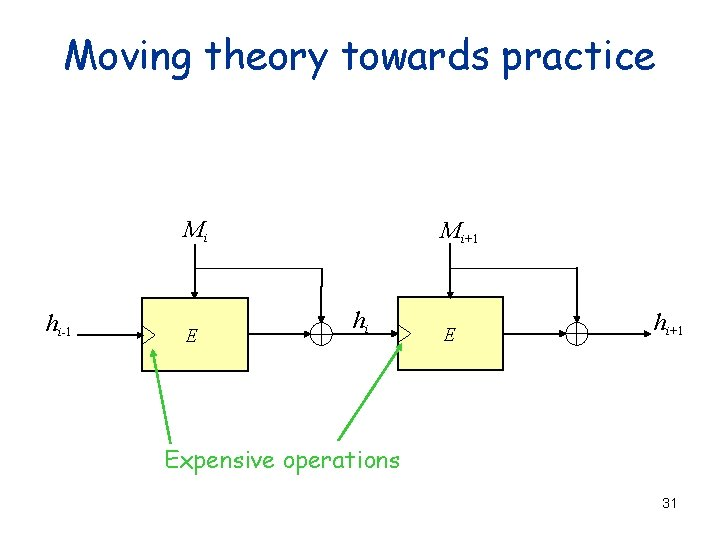Moving theory towards practice Mi hi-1 E Mi+1 hi E hi+1 Expensive operations 31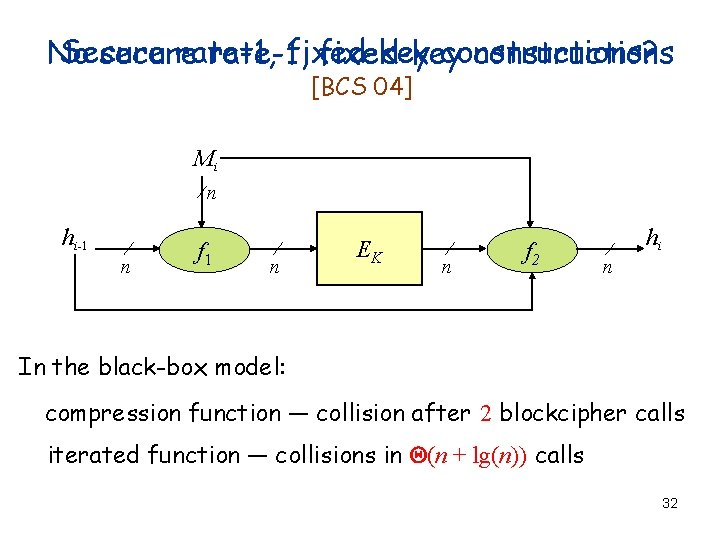Secure rate-1, fixed-key constructions? No secure rate-1, fixed-key constructions [BCS 04] Mi n hi-1 n f 1 n EK n f 2 hi n In the black-box model: compression function — collision after 2 blockcipher calls iterated function — collisions in Q(n + lg(n)) calls 32Research project #3 Find secure, fixed-key, rate < 1, iterated constructions (some progress being made) 33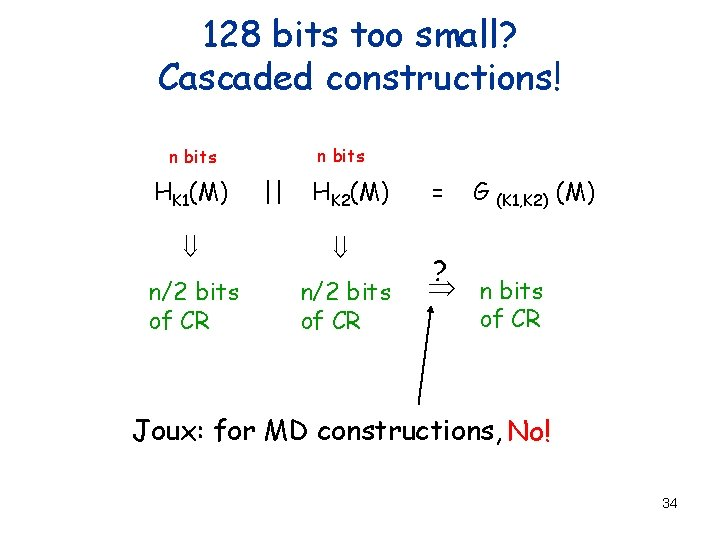128 bits too small? Cascaded constructions! n bits HK 1(M) || HK 2(M) ß ß n/2 bits of CR = G (K 1, K 2) (M) ? Þ n bits of CR Joux: for MD constructions, No! 34Multicollisions M 1 Mm M 2 n f IV n h 1 n f h 2 … hm-1 f hm = H (M) For m(2 n/2) work, we can make 2 m messages that collide 35Collisions in cascaded constructions 160 bits For G (K 1, K 2) 160 bits (M) = HK 1(M) || HK 2(M) : 1. Create 281 -way multicollision under HK 1 2. Hash these messages under HK 2 Collision in G for work O(280) << O(2160) 36What about MDC-2? Mi hi-1 E gi-1 E hi gi 37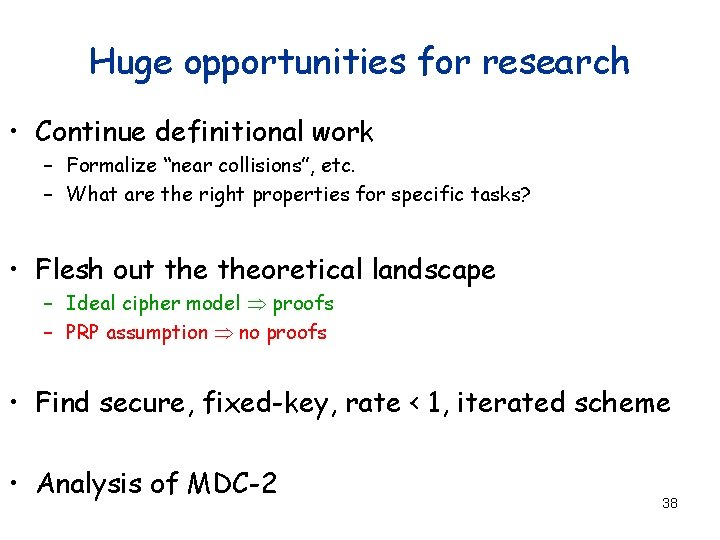Huge opportunities for research • Continue definitional work – Formalize “near collisions”, etc. – What are the right properties for specific tasks? • Flesh out theoretical landscape – Ideal cipher model Þ proofs – PRP assumption Þ no proofs • Find secure, fixed-key, rate < 1, iterated scheme • Analysis of MDC-2 383940